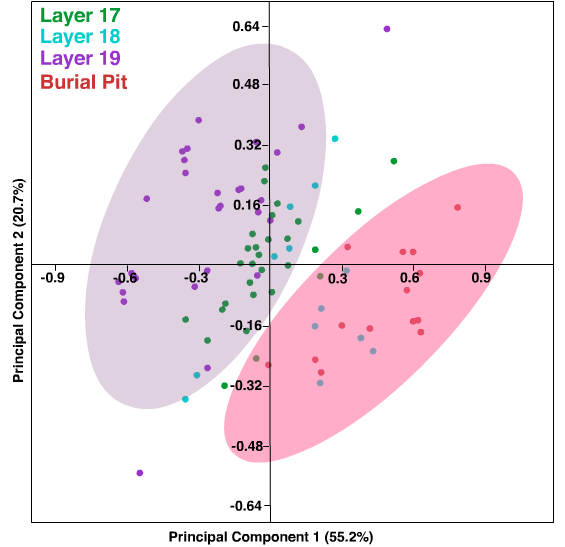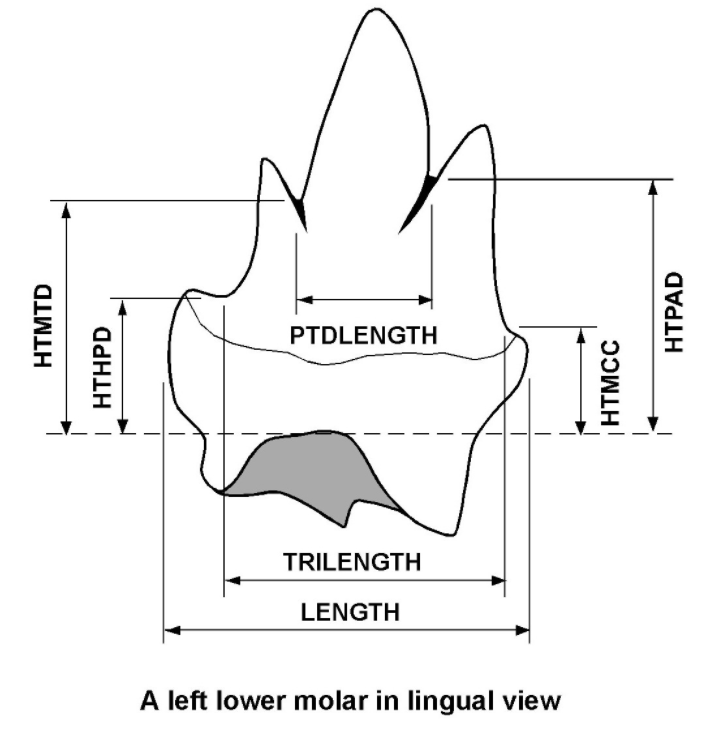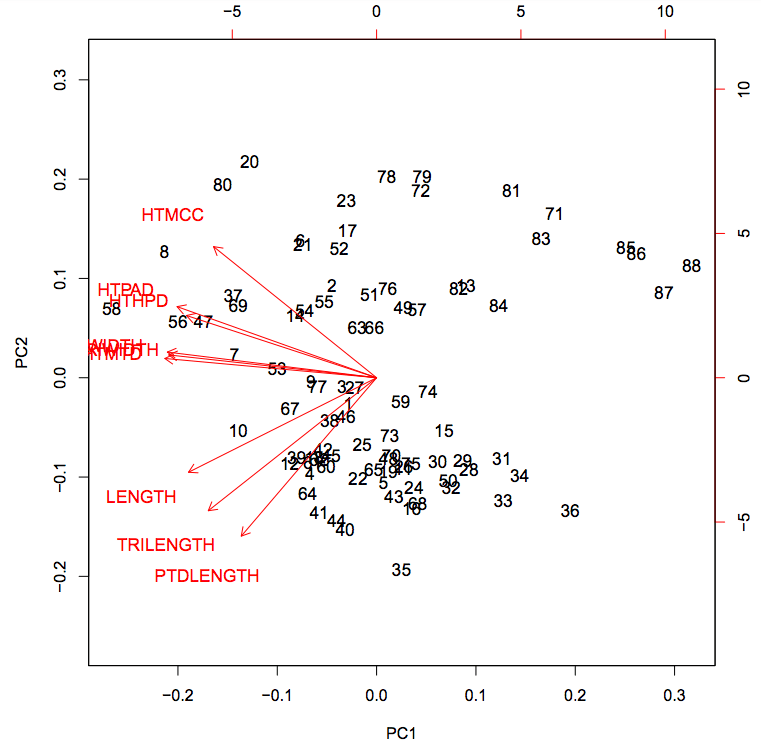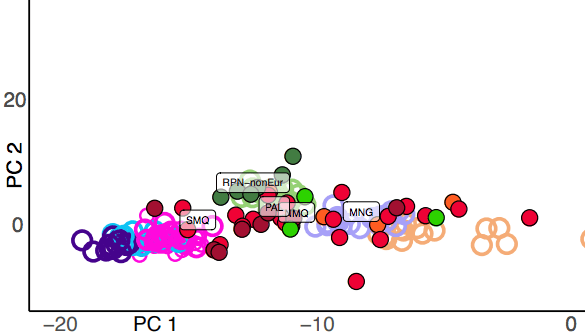# Three Examples and some Theory

Very often, especially in applications to the life sciences, useful low-dimensional projections exist and allow humans to grasp a data set that would otherwise be inscrutable.

Tony Phillips
Stony Brook University

### Introduction

Principal component analysis (PCA), an algorithm for helping us understand large-dimensional data sets, has become very useful in science (for example, a search in Nature for the year 2020 picks it up in 124 different articles). Human minds are good at recognizing patterns in two dimensions and to some extent in three, but are essentially useless in higher dimensions. The goal of PCA is to produce the most useful possible 2 or 3-dimensional projection of a high-dimensional data set—most useful in that the smallest amount of information is lost by the projection. One can construct artificial examples where all the dimensions must be considered, but very often, especially in applications to the life sciences, useful low-dimensional projections exist and allow humans to grasp a data set that would otherwise be inscrutable. In this column we will look at PCA in three recently published applications (to anthropology and paleontology), and go through the mathematics that makes it work.

### Example 1. Distinguishing nearby soil samples in a 78,000-year-old burialIn this PCA, 13-dimensional data from some 80 soil samples are projected into the plane spanned by their two principal components. The projection shows a clear distinction (highlighted by the superimposed 95% confidence ellipses) between samples from the burial pit (red dots) and samples (purple dots) from outside the pit at the same level (Layer 19) of the excavation. Image adapted from Nature, 593, 95-100, Extended Data Figure 4

Earliest known human burial in Africa was published in Nature on May 5, 2021. The authors are an international team of 36 led by María Martinón-Torres, Nicole Boivin and Michael Petraglia. In documenting the 78,000-year-old grave of a child in Panga ya Saidi, Kenya, they needed to establish that there had actually been a burial; they did this in part by showing that the earth surrounding the skeleton was different from the surrounding earth at that level (layer 19) in the archaeological excavation, i.e. that a pit had been dug to receive the child’s corpse. “The burial fill is a mix of ferruginous silt and sand, compositionally similar to the top of layer 18 and the base of layer 17, and different from layer 19, in which the pit was excavated.” They confirmed the difference by measuring the concentrations of 13 chemical elements occurring in the burial fill and in those three layers. To visuaize their ensemble of measurements (80 data points, each with 13 components), “[w]e performed PCA of EDXRF concentrations for the thirteen major, minor, and trace elements more frequently detected (Si, K, Ca, Ti, Mn, Fe, Zn, Ga, As, Rb, Y, Zr and Ba).” As they report, “The burial pit samples markedly differ from layer 19.”

### The basic mathematics

In the “Earliest … burial” example, PCA gives a useful 2-dimensional representation of a cloud of points in a 13-dimensional space. (Astronomers describe the general procedure as “finding the principal axes of inertia of the cloud of samples about their mean in feature space.”) The a priori unlikely combination of basic elements of statistics, multivariable calculus and linear algebra that makes it work will be outlined in this section.

To be specific, suppose the data set consists of $n$ measurements taken on $M$ samples; geometrically it would be a set of $M$ points distributed in $n$-dimensional space, where each sample is a point and its $n$ measurements are its $n$ coordinates.

• To fix notation, let measurements $x_1, \dots, x_n$ be taken on $M$ samples $s_1, \dots, s_m$.

• For each $x_i$ we calculate the average, or mean

$$\overline{x_i} = \frac{1}{M}\sum_{j=1}^M x_i(s_j).$$

• Then for each $x_i$ we calculate the variance: this is the average of the square of the difference between the $x_i$-measurements and the mean: $${\rm Var}(x_i) = \frac{1}{M}\sum_{k=1}^M (x_i(s_k)-\overline{x_i})^2.$$

• The variance ${\rm Var}(x_i)$ estimates the spread of $x_i$. Squaring the differences puts deviations to the right or the left of the mean on the same footing; it also gives more weight to the larger differences (the “outliers”). (The standard deviation, a more common measure of spread, is defined as the square root of the variance.)

• For each pair $(x_i, x_j)$ of measurements we calculate the covariance $${\rm Cov}(x_i, x_j) = \frac{1}{M}\sum_{k=1}^M (x_i(s_k)-\overline{x_i})(x_j(s_k)-\overline{x_j}).$$ Note that ${\rm Cov}(x_i, x_i)={\rm Var}(x_i)$.

The various covariances are naturally displayed in the covariance matrix

$${\bf Cov} ={\bf Cov}(x_1,\cdots, x_n)=\left (\begin{array}{cccc}{\rm Cov}(x_1,x_1)& {\rm Cov}(x_1,x_2)&\cdots&{\rm Cov}(x_1,x_n)\\ {\rm Cov}(x_2,x_1)&{\rm Cov}(x_2,x_2) &\cdots &{\rm Cov}(x_2,x_n)\\ \vdots &\vdots&\vdots\\ {\rm Cov}(x_n,x_1)&{\rm Cov}(x_n,x_2)&\cdots&{\rm Cov}(x_n,x_n) \end{array} \right ).$$

Note that since ${\rm Cov}(x_i, x_j) = {\rm Cov}(x_j, x_i)$ the matrix ${\bf Cov}$ is symmetric. This fact will be very important when we study its algebraic properties.

So much for the statistics. The next calculations will be simpler to write if we assume our initial measurements have been centered (by subtracting from each one its original mean), so that each one has mean equal to zero. So now ${\rm Cov}(x_i, x_j) = \frac{1}{M}\sum_{k=1}^M x_i(s_k)x_j(s_k),$ for all $i,j=1,\dots,n$.

PCA starts with finding the direction in $n$-space (measurement-space) along which the data are maximally spread out, so that the clustering and spacing of the data points along that direction carry the most information. A direction is given by a unit vector ${\bf a}$ with components $a_1, \dots, a_n$, and the measurement in this direction is the linear combination $y=a_1 x_1 + \dots + a_n x_n$. Since the $x_i$ all have mean zero, the same must hold for $y$, so it is automatically centered. “Maximally spread out” in our context means having the greatest variance ${\rm Var}(y) = \frac{1}{M}\sum_{j=1}^M y(s_j)^2$.

So we want to find the components $a_1, \dots, a_n$ leading to the maximum of ${\rm Var}(y) = {\rm Var}(a_1 x_1 + \dots + a_n x_n)$, subject to the constraint $a_1 ^2 +\cdots +a_n^2=1$. Geometrically, we are maximizing ${\rm Var}(y)$ on the surface of the unit sphere in $n$-space. The way we learn to do this in multivariable calculus is to look for the points on the sphere $a_1 ^2 +\cdots +a_n^2=1$ where the gradient of ${\rm Var}(y)$ is perpendicular to the surface of the sphere or, equivalently, parallel to the gradient of the function $a_1 ^2 +\cdots +a_n^2$, which is $(2a_1, \dots, 2a_n)$. One of these points will be the maximum. (The  gradient of a function $f$ of $n$ variables $x_1, \dots, x_n$ is the vector $(\partial f/\partial x_1, \dots, \partial f/\partial x_n)$. It points in the direction of steepest increase.)

Numerically, the statement that the gradients of ${\rm Var}(y)$ and $a_i ^2 +\cdots +a_n^2$ are parallel means that there is a non-zero scalar $\lambda$ such that one is $\lambda$ times the other (this is the basis of the method of  Lagrange multipliers):

$$\frac{\partial {\rm Var}(y)}{\partial a_1}= 2\lambda a_1$$

$$~~~~~~~~~~~~~~~~\vdots ~~~~~~~~~~~~~~~~(*)$$

$$\frac{\partial {\rm Var}(y)}{\partial a_n} = 2\lambda a_n.$$

Let us calculate $\partial {\rm Var}(y)/\partial a_j$.

$$\frac{\partial}{\partial a_j}{\rm Var}(y) = \frac{\partial}{\partial a_j}{\rm Var}(a_1 x_1 + \dots + a_n x_n).$$

Remembering that the $x_i$ are centered,

$${\rm Var}(a_1 x_1 + \dots + a_n x_n)= \frac{1}{M}\sum_{k=1}^M (a_1 x_1(s_k) + \dots + a_n x_n(s_k))^2$$

so by the chain rule

$$\frac{\partial}{\partial a_j}{\rm Var}(y)= \frac{1}{M} \sum_{k=1}^M [2(a_1 x_1(s_k) + \dots + a_n x_n(s_k))\cdot x_j(s_k)]$$

$$= 2a_1{\rm Cov}(x_1,x_j) + \cdots + 2a_n {\rm Cov}(x_n,x_j).$$

Equations $(*)$ then imply that for each $j$ between 1 and $n$,

$$2a_1 {\rm Cov}(x_1,x_j) + \cdots + 2a_n {\rm Cov}(x_n,x_j) = 2\lambda a_j.$$

Here is where linear algebra makes a miraculous intervention. Cancelling the 2s, and remembering that  ${\rm Cov}(x_i,x_j) = {\rm Cov}(x_j,x_i)$, these equations together can be rewritten as

$$\left ( \begin{array}{cccc} {\bf Cov}(x_1,x_1)& {\bf Cov}(x_1,x_2)& \cdots &{\bf Cov}(x_1,x_n)\\ {\bf Cov}(x_2,x_1)&{\bf Cov}(x_2,x_2) &\cdots &{\bf Cov}(x_2,x_n)\\ \vdots&\vdots&\vdots&\vdots\\ {\bf Cov}(x_n,x_1)&{\bf Cov}(x_n,x_2)&\cdots&{\bf Cov}(x_n,x_n) \end{array} \right ) \left( \begin{array}{c}a_1\\ a_2\\ \vdots\\ a_n \end{array} \right )= \lambda \left( \begin{array}{c}a_1\\ a_2\\ \vdots\\ a_n \end{array} \right ).~(**)$$

We say that the column vector ${\bf a}$  is an eigenvector for the covariance matrix ${\bf Cov}$, with eigenvalue $\lambda$.

Finding the eigenvalues and eigenvectors for the $n\times n$ matrix ${\bf Cov}$ is a standard set of calculations in linear algebra, which I will summarize here.

• The eigenvalues of ${\bf Cov}$ are defined as the roots of the degree-$n$ polynomial $\det({\bf Cov}-\lambda {\rm I})=0$, where ${\rm I}$ is the $n\times n$ identity matrix; but for large $n$ this definition is not practical: eigenvectors and eigenvalues are calculated by the Lagrange multiplier method as above. In applications to the life sciences, where there are not likely to be hidden symmetries in the problem, the eigenvalues will be all distinct; furthermore, since ${\bf Cov}$ is a symmetric matrix these are all real numbers, and can therefore be ordered $\lambda_1 > \lambda_2 > \cdots > \lambda_n$.

• For each index $j$, the vector equation ${\bf Cov} \cdot {\bf a} = \lambda_j {\bf a}$ has a non-zero solution, unique up to a scalar multiple, so unique up to sign if we fix its length to be 1. This solution, call it ${\bf a}_j$, is the eigenvector corresponding to $\lambda_j$.

• (For future reference, here is a useful fact: because the matrix is symmetric, eigenvectors corresponding to different eigenvalues are perpendicular.)

In particular the column vector ${\bf a}_1$, with components $a_{11}, \dots, a_{1n}$, corresponding to the maximum eigenvalue $\lambda_1$, gives us the answer to our original question: the direction in measurement-space along which the data are maximally spread out. Notice that the indeterminacy in the sign of ${\bf a}_1$ does not affect its direction. In PCA, the quantity $y_1 = a_{11}x_1 + \dots + a_{1n}x_n$ is called PC1, the first principal component.

What is this maximum variance? The answer involves some calculation.

Remember that for $\displaystyle{y_1 = a_{11}x_1 +\dots + a_{1n}x_n}$,
$${\rm Var}(y_1) ={\rm Var}(a_{11} x_1 + \dots + a_{1n} x_n)= \frac{1}{M}\sum_{k=1}^M (a_{11} x_1(s_k) + \dots + a_{1n} x_n(s_k))^2$$
so
$${\rm Var}(y_1) =\frac{1}{M}\sum_{k=1}^M \sum_{i=1}^n\sum_{j=1}^n a_{1i}a_{1j}x_i(s_k)x_j(s_k)$$

$$= \sum_{i=1}^n\sum_{j=1}^n a_{1i}a_{1j}\frac{1}{M}\sum_{k=1}^M x_i(s_k)x_j(s_k) = \sum_{i=1}^n\sum_{j=1}^n a_{1i}a_{1j} {\rm Cov}(x_i,x_j)$$

$$= (a_{11} ~ a_{12}~ \cdots ~a_{1n}) \left ( \begin{array}{cccc} {\rm Cov}(x_1,x_1)& {\rm Cov}(x_1,x_2)&\cdots&{\rm Cov}(x_1,x_n)\\ {\rm Cov}(x_2,x_1)&{\rm Cov}(x_2,x_2) &\cdots &{\rm Cov}(x_2,x_n)\\ \vdots&\vdots&\vdots&\vdots\\ {\rm Cov}(x_n,x_1)&{\rm Cov}(x_n,x_2)&\cdots&{\rm Cov}(x_n,x_n) \end{array} \right ) \left( \begin{array}{c}a_{11}\\ a_{12}\\ \vdots\\ a_{1n} \end{array} \right )$$
$$=(a_{11} ~ a_{12}~ \cdots ~a_{1n})~ \lambda_1 \left( \begin{array}{c}a_{11}\\ a_{12}\\ \vdots\\ a_{1n} \end{array} \right ) = \lambda_1 (a_{11}^2 + a_{12}^2 + \cdots a_{1n}^2) = \lambda_1.$$

Amazingly enough, the variance of the linear combination $y_1 = a_{11}x_1 + \dots + a_{1n}x_n$ using the coefficients of ${\bf a}_1$ is exactly the corresponding eigenvalue $\lambda_1$.

Once we have found ${\bf a}_1$, we go on to look for the next most useful direction in which to sort the data. We look in the hyperplane (in measurement-space) perpendicular to ${\bf a}_1$ and repeat the analysis. As before we want to maximize ${\rm Var}(y) = {\rm Var}(b_1 x_1 + \dots + b_n x_n)$; subject to the constraint $b_1 ^2 +\cdots +b_n^2=1$; now we add the additional constraint $b_1a_{11} + \cdots + b_na_{1n} = 0$. The solution will be a point satisfying $b_1 ^2 +\cdots +b_n^2=1$ where the gradient of ${\rm Var}(y)$ is parallel to the gradient of $b_i ^2 +\cdots +b_n^2$ and lies in the plane $b_1a_{11} + \cdots + b_na_{1n} = 0$. As before, the solution is an eigenvector of the covariance matrix; the condition $b_1a_{11} + \cdots + b_na_{1n} = 0$ eliminates ${\bf a}_1$, leaving ${\bf a}_2$ as the direction maximizing variance; $y_2 = a_{21}x_1 + \cdots a_{2n}x_n$ will be PC2, the second principal component, with variance calculated as above to be $\lambda_2$.

Some more linear algebra: equation $(**)$ specialized to the vector ${\bf a}_i$ can be written in matrix notation ${\bf Cov}\cdot{\bf a}_i = \lambda_i{\bf a}_i$. Amalgamating the $n$ column vectors ${\bf a}_1, \dots {\bf a}_n$ into a matrix ${\bf A}$ will give ${\bf Cov}\cdot{\bf A} = {\bf A}\cdot{\bf \Lambda}$, where ${\bf \Lambda}$ is the diagonal matrix with entries $\lambda_1, \dots, \lambda_n$. Now ${\bf A}$, being made up of orthogonal columns of length 1, is an orthogonal matrix; its inverse is easy to calculate: it is the transpose ${\bf A}^{\top}$. Multiplying both sides of the last equation on the left by ${\bf A}^{\top}$ gives ${\bf A}^{\top}\cdot{\bf Cov}\cdot{\bf A} = {\bf A}^{\top}\cdot {\bf A}\cdot {\bf \Lambda}= {\bf \Lambda}$. On the other hand, ${\bf A}^{\top}\cdot {\bf Cov}\cdot{\bf A}$, being similar to ${\bf Cov}$, has the same trace ${\rm Cov}(x_1, x_1) + \cdots + {\rm Cov}(x_n, x_n)$, which then must be equal to the trace of ${\bf \Lambda}$, i.e. $\lambda_1 + \cdots + \lambda_n$. The sum of the eigenvalues is equal to the total variance. Often in applications to the life sciences the first two eigenvalues together account for a large proportion of the variance, and their associated principal components give a satisfactory two-dimensional representation of the entire $n$-dimensional panorama of the measurements (in our first two examples they account for 76% and 95.5%, respectively, of the total variance). Often also the third principal component is calculated to further refine the picture.

To complete the picture the $M$ points in $n$-space corresponding to the original measurements can be mapped into the (CP1, CP2)-plane using the matrix ${\bf A}$: $y_1(s_k) = a_{11}x_1(s_k)+\cdots+a_{1n}x_n(s_k)$, $y_2(s_k) = a_{21}x_1(s_k)+\cdots+a_{2n}x_n(s_k)$, or into (CP1, CP2, CP3)-space by using also $y_3(s_k) = a_{31}x_1(s_k)+\cdots+a_{3n}x_n(s_k)$.

## Example 2. Sorting Kuehneotherium teethKuehneotherium (right) and its 200-million- years-ago contemporary Morganucodon are imagined by John Sibbick foraging for insects in what is now part of Wales. The painting, which appeared on the cover of Nature, is reproduced courtesy of Pamela Gill.

This example, which comes from a review article on PCA by Ian T. Jolliffe and Jorge Cadima in  Philosophical Transactions of the Royal Society A, “examines a dataset consisting of nine measurements on 88 fossil teeth from the early mammalian insectivore Kuehneotherium . …  Kuehneotherium is one of the earliest mammals and remains have been found during quarrying of limestone in South Wales, UK. The bones and teeth were washed into fissures in the rock, about 200 million years ago, and all the lower molar teeth used in this analysis are from a single fissure. However, it looked possible that there were teeth from more than one species of  Kuehneotherium in the sample.” The teeth came from the collection of the Natural History Museum London, and the measurements were made by Pamela G. Gill, School of Earth Sciences, Bristol. Her nine measurements are identified in the next figure.The nine parameters measured by Pamela Gill on each Kuehneotherium tooth. Image courtesy of Pamela Gil.

Cadima and Jolliffe ran a PCA of a subset Gill’s measurements (all the teeth from one of the fissures), and obtained the plot shown here.Cadima and Jolliffe’s PCA of the Kuehneotherium dataset. The original 88 data points have been projected onto the (PC1, PC2) plane, along with vectors corresponding to the nine original measurements. Image courtesy of Jorge Cadima.

They remark: “The first two PCs account for 78.8% and 16.7%, respectively, of the total variation in the dataset, so the two-dimensional scatter-plot of the 88 teeth given by [the figure] is a very good approximation to the original scatter-plot in nine-dimensional space.” Furthermore “All of the loadings in the first PC have the same sign, so it is a weighted average of all variables, representing ‘overall size’. In [the figure], large teeth are on the left and small teeth on the right. The second PC has negative loadings for the three length variables and positive loadings for the other six variables, representing an aspect of the ‘shape’ of teeth. Fossils near the top of [the figure] have smaller lengths, relative to their heights and widths, than those towards the bottom.”

They conclude: “The relatively compact cluster of points in the bottom half of [the figure] is thought to correspond to a species of Kuehneotherium, while the broader group at the top cannot be assigned to Kuehneotherium, but to some related, but as yet unidentified, animal.”

## When the measurement space has very high dimension

In applications to genetics or to image analysis (where each pixel is a “measurement”) the number $n$ of measurements is often enormous compared to the number $M$ of samples. Following the CPA algorithm sketched above would mean dealing with an intractably large matrix.

Now this problem is only potentially $n$-dimensional, because all we have to work with are data from $M$ points. One way to conceptualize it is to think of each of the aggregate measurement results $x_i$ as a vector ${\bf x}_i$ with components $x_i(s_1), \dots x_i(s_M)$. Now we have $n$ vectors in an $M$-dimensional space, with $n>M$, so there is a set of (at most) $M$ linearly independent vectors which give all the ${\bf x}_i$ by linear combination. Suppose for simplicity there are exactly $M$, say ${\bf z}_1,\dots, {\bf z}_M$. Then our problem of finding a variance-maximizing linear combination $y=a_1x_1 + \dots +a_nx_n$, i.e. ${\bf y} = a_1{\bf x}_1 + \dots +a_n{\bf x}_n$ reduces to finding the much smaller linear combination ${\bf y} = c_1{\bf z}_1 + \dots +c_M{\bf z}_M$ maximizing variance. But how do we identify ${\bf z}_1,\dots, {\bf z}_M$?

Again, linear algebra has just the tools we need. With the same notation as above, let us compute for every $i,j$ between 1 and $M$ the quantity $\frac{1}{n}(x_1(s_i)x_1(s_j)+x_2(s_i)x_2(s_j) + \cdots + x_n(s_i)x_n(s_j))$. These numbers fit in a new, $M\times M$, matrix we can call ${\bf Voc}$ to distinguish it from ${\bf Cov}$ defined above. We calculate the eigenvectors ${\bf b}_1, \dots, {\bf b}_M$ and corresponding eigenvalues $\lambda_1, \dots, \lambda_M$ for ${\bf Voc}$ as we did before.

There is a useful relation between ${\bf Cov}$ and ${\bf Voc}$. Suppose we call ${\bf X}$ the matrix of all the measurements of all the samples, so ${\bf X}_{ij} = x_i(s_j)$, and its transpose ${\bf X}^{\top}_{ij} = {\bf X}_{ji} = x_j(s_i)$. The matrix ${\bf X}$ has $M$ rows and $n$ columns, vice-versa for ${\bf X}^{\top}$. The matrix product ${\bf X}\cdot {\bf X}^{\top}$ is defined by $[{\bf X}\cdot {\bf X}^{\top}]_{ij} = {\bf X}_{i1} {\bf X}^{\top}_{1j} + \cdots + {\bf X}_{iM} {\bf X}^{\top}_{Mj} = x_i(s_1)x_j(s_1) + \cdots + x_i(s_M)x_j(s_M)$ $= M {\rm Cov}(x_1, x_j)$ whereas $[{\bf X}^{\top}\cdot {\bf X}]_{ij} = {\bf X}^{\top}_{i1} {\bf X}_{1j} + \cdots + {\bf X}^{\top}_{in} {\bf X}_{nj} = x_1(s_i)x_1(s_j) + \cdots + x_n(s_i)x_n(s_j)$ $=n {\rm Voc}(s_i,s_j)$ So ${\bf X}\cdot {\bf X}^{\top} = n {\bf Cov}$, $n$ times the intractable matrix we are interested in, and ${\bf X}^{\top}\cdot {\bf X} = M {\bf Voc}$, $M$ times its smaller cousin. Now a little more linear algebra:

• ${\bf X}^{\top}\cdot {\bf X}$ and ${\bf X}\cdot {\bf X}^{\top}$ have the same non-zero eigenvalues, $\lambda_1, \dots, \lambda_M$
• For each eigenvector ${\bf b}_i$ of ${\bf X}^{\top}\cdot {\bf X}$, the vector ${\bf X}\cdot {\bf b}_i$ is an eigenvector for ${\bf X}\cdot {\bf X}^{\top}$ with the same eigenvalue $\lambda_i$.

The proofs of these a priori unlikely statements are immediate: If there is a non-zero vector ${\bf b}$ with ${\bf X}^{\top}\cdot {\bf X}\cdot {\bf b}=\lambda{\bf b}$, then ${\bf X}\cdot {\bf X}^{\top}\cdot {\bf X}\cdot {\bf b} =\lambda{\bf X}\cdot {\bf b}$, so ${\bf X}\cdot {\bf b}$ is an eigenvector for ${\bf X}\cdot {\bf X}^{\top}$ with eigenvalue $\lambda$.

Now if ${\bf b}$ is an eigenvector of the matrix ${\bf A}$ with eigenvalue $\lambda$, then ${\bf b}$ will also be an eigenvector of $k$ times ${\bf A}$, with eigenvalue $k\lambda$ (since $k{\bf A}\cdot {\bf b} = k({\bf A}\cdot {\bf b}) = k(\lambda {\bf b})=(k\lambda) {\bf b}$). So once we know the eigenvectors and eigenvalues for the smaller matrix ${\bf Voc}$, we can retrieve the eigenvectors and eigenvalues for ${\bf Cov}$, and complete our PCA.

The switch to the transpose makes examples like the next one possible.

## Example 3. Parsing Native American genes in Polynesian ancestry

“Native American gene flow into Polynesia predating Easter Island settlement” was published in Nature, July 8, 2020. The authors are a team of 32 led by Alexander G. Ioannidis (Stanford), Javier Blanco-Portillo and Andrés Moreno-Estrada (both at LANGEBIO, Irapuato, Mexico). They begin: “The possibility of voyaging contact between prehistoric Polynesian and Native American populations has long intrigued researchers.” They mention Thor Heyerdahl’s controversial suggestion “that prehistoric South American populations had an important role in the settlement of east Polynesia and particularly of Easter Island (Rapa Nui),” and that the matter is still “hotly contested.” No longer, presumably: “We find conclusive evidence for prehistoric contact of Polynesian individuals with Native American individuals (around AD 1200)… Our analyses suggest strongly that a single contact event occurred in eastern Polynesia, before the settlement of Rapa Nui, between Polynesian individuals and a Native American group most closely related to the indigenous inhabitants of present-day Colombia.”

Part of their evidence is a detailed analysis of the ancestry of present-day inhabitants of Rapa Nui. They split them into two groups: those who have European ancestry and those who do not. They present a PCA comparing these two groups (along with other Pacific islanders) with a spectrum of present-day Native American inhabitants of Central and South America. It shows that the Rapa Nui inhabitants with some European ancestry group with populations in the South of Chile (Mapuche, Pehuenche, Huiiliche, Chilote) while those with no European ancestry group with the Zenu, a population in Colombia, at the opposite end of South America.Part of Supplemental Figure 14 from {\em Nature} {\bf 583}, 572-577 (2020), used with permission. For legibility I have cut the PC1 axis in two and have suppressed the top of the range of PC2. The color key is shown separately below.Color key for the PCA by Ioannides et al.

The caption in Nature gives more detail:  “Our new weighted ancestry-specific SVD-completed principal component analysis (PCA) applied to the Native American component from Pacific islanders and reference individuals from the Americas. The Native American ancestries of reference individuals are plotted with open circles. The Native American ancestries of Pacific islanders are represented as solid points. … In addition, labels are plotted for the location of the allele frequency vector created from aggregating all Native American ancestry haplotypes in each island population. The Rapanui are split into two populations, those without European ancestry tracts (dark green) and those with high European and Native American ancestry (blue). The first principal component axis separates the reference Native Americans on a north-south axis, while the second principal component separates the Yamana of southern Patagonia. Pacific islanders’ Native American ancestry is seen to cluster with northern South American references with the exception of the Rapanui with high European ancestry, who cluster with the southern references (Chilean).”

Conversations with Folkert Tangerman were very helpful in the preparation of this column.

Updated 31 August in response to a comment from a reader.

1.About 11 lines before the start of Example 2, you say that “a diagonal matrix commutes”. Is this true? Why?

BTW, an excellent article!

2.Thanks. A diagonal matrix does not commute in general.
And Cov . A is A . Lambda [not Lambda . A] so the
commutation was not necessary. I’ll try to get this corrected. Tony

3.In the line where the covariance is defined, shouldn’t the last index be a “j” so that the term is avg(xj) ?

1.Thanks for catching this! We’ve updated the article.